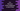# How to find the sum of two distances in inch and feet in C++## How to find the sum of two distances in inch and feet in C++:

In this post, we will write one C++ program to find the sum of two distance values. Each distance value is represented in feet and inches. We know that, 1 feet = 12 inches. So, our program will do the conversion and add the two distance values.

We will use structure to store the distance inch and feet values and to add them. Using a structure makes it easy to store and do the calculation.

### C++ program:

Let’s take a look at the program:

``````#include <iostream>
using namespace std;

struct Distance
{
int feet;
int inch;
};

int main()
{
Distance first, second, sum;

cout << "Enter the first distance in feet and inch :" << endl;
cin >> first.feet >> first.inch;

cout << "Enter the second distance in feet and inch :" << endl;
cin >> second.feet >> second.inch;

sum.feet = first.feet + second.feet;
sum.inch = first.inch + second.inch;

if (sum.inch > 12)
{
sum.feet += sum.inch/12;
sum.inch  = sum.inch % 12;
}

cout << "Sum of distances : " << sum.feet << " feet," << sum.inch << " inches" << endl;
}``````

### Explanation:

In this program,

• Distance is a structure defined to store a distance in feet and inches. It can hold these values as integer.
• We are taking the inputs of the first and second distance in feet and inch from the user and storing them in first and second Distance variables.
• We are storing the sum of feet and inch values of both first and second to the variable sum.
• We are also checking if the value inch is more than 12. If it is, we are incrementing the value of feet by sum.inch/12 and decrementing the value of inch of sum by sum.inch % 12.
• Finally, we are printing the values of sum.

### Output:

This program will print output as like below:

``````Enter the first distance in feet and inch :
10 20
Enter the second distance in feet and inch :
9 15
Sum of distances : 21 feet,11 inches``````

If we add the feet and inches, then it becomes 19 feet and 35 inches. 35 inches is equal to 2 feet, 11 inches. So, the sum becomes 21 feet, 11 inches.